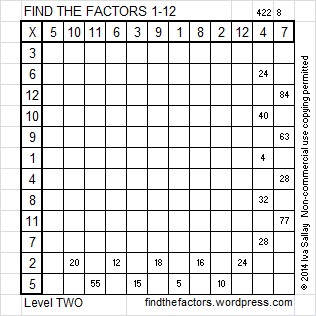# 135 and Level 2

135 is a composite number. 135 = 1 x 135, 3 x 45, 5 x 27, or 9 x 15. Factors of 135: 1, 3, 5, 9, 15, 27, 45, 135. Prime factorization: 135 = 3 x 3 x 3 x 5, which can also be written 135 = 3³ x 5.1 + 3 + 5 = 9. Nine is a multiple of both 3 and 9, so this quick divisibility trick lets us know that both 3 and 9 are factors of 135.

135 = 1 x 3³ x 5¹. Click 135 to learn two other ways to have 1, 3, 5 on both sides of the equal sign.

135 is never a clue in the FIND THE FACTORS puzzles.Excel file with puzzles and the previous week’s factor solutions: 12 Factors 2014-06-02This site uses Akismet to reduce spam. Learn how your comment data is processed.